## SOCNET@LISTS.UFL.EDU

#### View:

 Message:[First|Previous|Next|Last] By Topic:[First|Previous|Next|Last] By Author:[First|Previous|Next|Last] Font: Proportional Font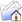LISTSERV Archives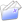SOCNET HomeSOCNET December 2005

Subject:Does p* contain p2?

From:Date:Thu, 15 Dec 2005 09:44:20 +0900

Content-Type:text/plain

Parts/Attachments: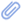text/plain (45 lines)
 ```***** To join INSNA, visit http://www.insna.org ***** I've read some papers about p* and one paper about p2. If I've read correctly, Equation 4 of reference 2 ;extends ;first p* (equation 1) to contain covariates. And p2 is 'the extension of p1 that doesn't assume dyadic independence and accounts for nodal & dyadic covariates.' Does p* (equation 4 in reference 2) contain p2? Because p* has p1 as submodels, p2's model equation is very similar to p*'s. If not, what is the unique features of p2? Thanks in advance for any comments. --- Equations --- Equation 1 of Reference 2 ; : ; P(X = x) ;= exp(theta' * ;t(x)) / c(theta) Equation 4 of Reference 2 ; : ; P(X = x) = exp(vec(x)' Z beta + sum of alpha_k * d_k(x) + theta' * t(x)) / c(alpha, beta, theta) ; (d_k : the number of individuals with exactly k links, Z : exogenous dyadic covariates) --- References --- 1. Logit models and logistic regressions for social networks : I An Introduction to markov graphs and p*. Stanley ;Wasserman... 2. statnet: An R package for the Statistical Analysis and Simulation of Social Networks, Mark S. Handcock... 3. p2: a random effects model with covariates for directed graphs. Marijtje A. J. van Duijn... Best Regards Se Kwon, Kim ------------- KAIST (Korean Advanced Institute of Science & Technology) Mathematics Student http://math.kaist.ac.kr _____________________________________________________________________ SOCNET is a service of INSNA, the professional association for social network researchers (http://www.insna.org). To unsubscribe, send an email message to [log in to unmask] containing the line UNSUBSCRIBE SOCNET in the body of the message.```

#### Search Archives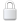Log In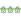Get Password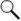Search Archives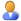Subscribe or Unsubscribe